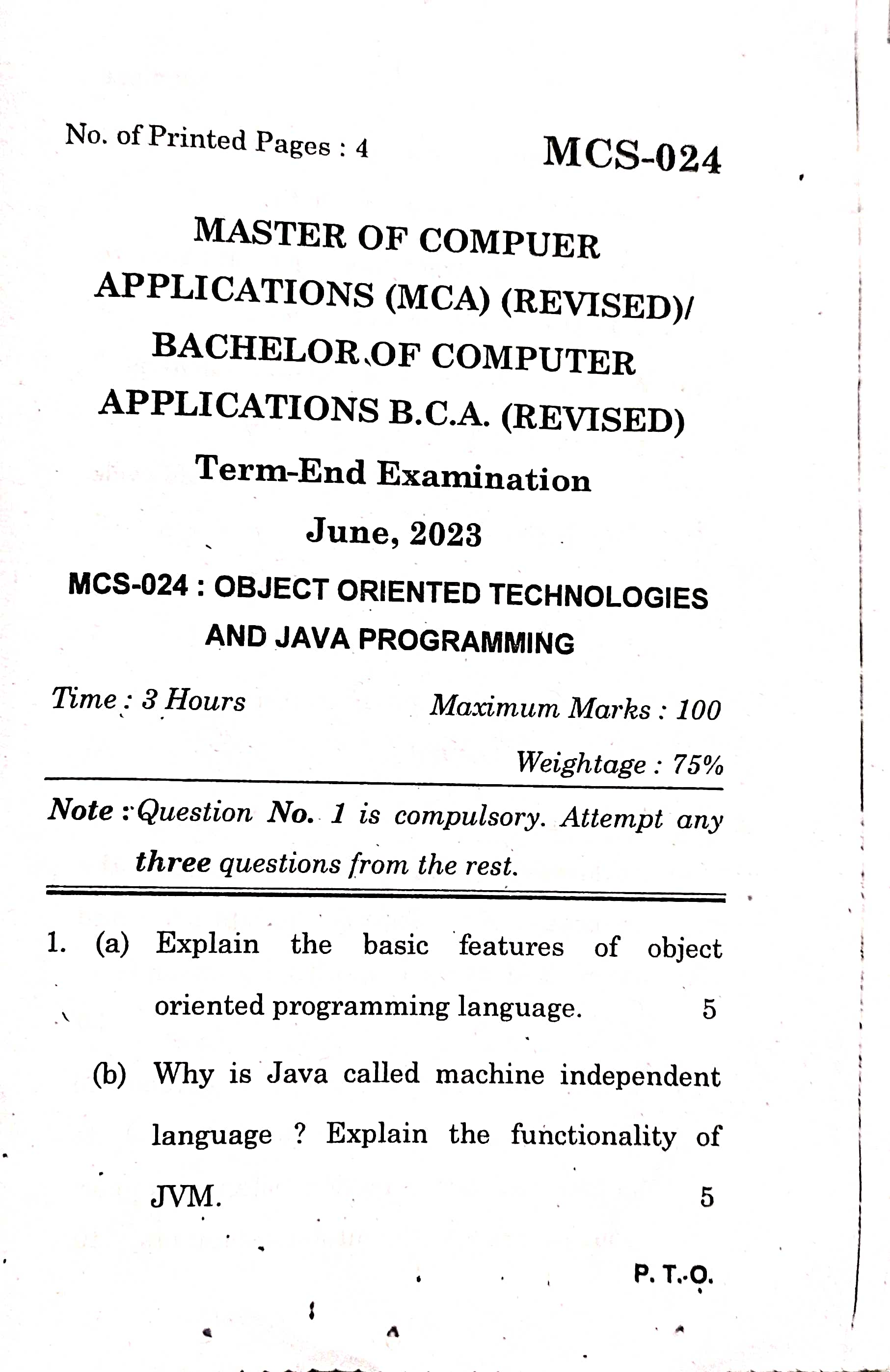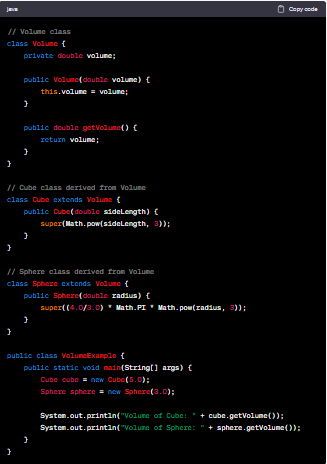# MCS024 : Question 4 (b) Java Program - Volume Calculation for Cube and Sphere Classes | June 2023 Paper Solution### Question 4(b) Write a java program to create a volume class. Derive cube and sphere classes from it. Define constructor for these three classes.

// Volume class
class Volume {
private double volume;

public Volume(double volume) {
this.volume = volume;
}

public double getVolume() {
return volume;
}
}

// Cube class derived from Volume
class Cube extends Volume {
public Cube(double sideLength) {
super(Math.pow(sideLength, 3));
}
}

// Sphere class derived from Volume
class Sphere extends Volume {
super((4.0/3.0) * Math.PI * Math.pow(radius, 3));
}
}

public class VolumeExample {
public static void main(String[] args) {
Cube cube = new Cube(5.0);
Sphere sphere = new Sphere(3.0);
System.out.println("Volume of Cube: " + cube.getVolume());
System.out.println("Volume of Sphere: " + sphere.getVolume());
}
}JAVA Program

In this program, we have a "Volume" class that represents the concept of volume. It has a private
"Volume" variable and a constructor that initializes the volume.

The "Cube" class is derived from the "Volume" class using the "extends" keyword. It has a constructor that takes the side length of the cube as a parameter. Inside the constructor, we call the "super()" method to invoke the constructor of the "Volume" class and calculate the volume of the cube by raising the side length to the power of 3.

The "Sphere" class is also derived from the "Volume" class and has a constructor that takes the radius of the sphere as a parameter. Again, we call the "super()" method to invoke the constructor of the "Volume" class and calculate the volume of the sphere using the formula "(4/3) * π * radius^3".

In the "main()" method, we create instances of the "Cube" and "Sphere" classes, passing the required parameters to their respective constructors. We then use the "getVolume()" method to retrieve the calculated volumes and display them on the console.

When you run this program, it will output:

Volume of Cube: 125.0
Volume of Sphere: 113.09733552923254

This demonstrates the usage of the "Volume" class and its derived classes, "Cube" and "Sphere", with constructor definitions for each class.